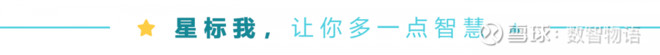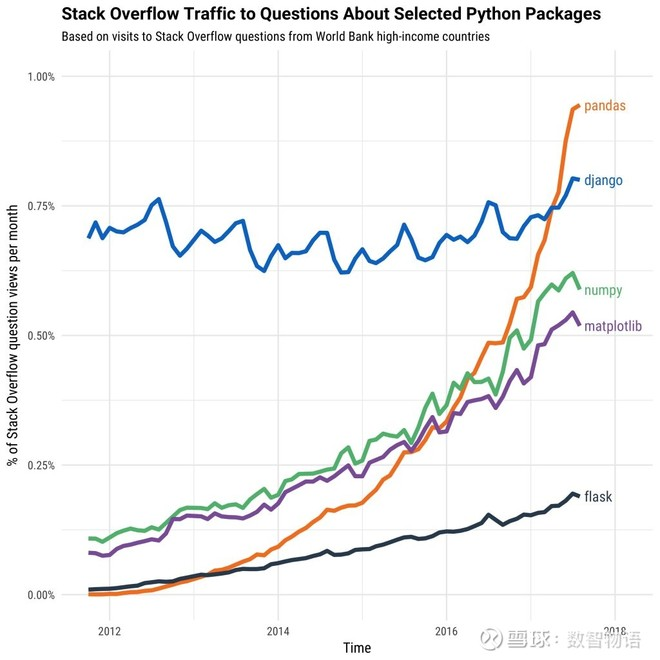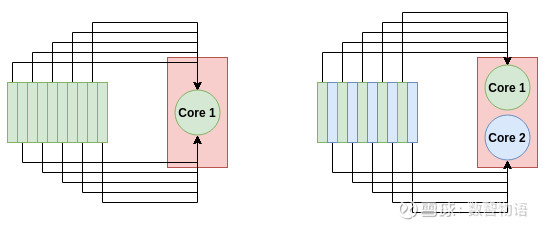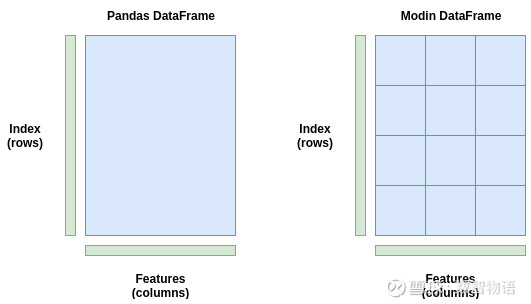# 一行代码将Pandas加速4倍Pandas是处理 Python 数据的首选库。它易于使用，并且在处理不同类型和大小的数据时非常灵活。它有大量的函数，使得操纵数据变得轻而易举。Modin是一个新的库，通过在系统所有可用的 CPU 核上自动分配计算来加速 pandas。有了它，对于任何尺寸的 pandas 数据数据集，Modin 声称能够以 CPU 内核的数量得到近乎线性的加速。

01Modin 如何用 Pandas 并行计算panda的DataFrame(左)存储为一个块，只发送到一个CPU核。Modin的DataFrame(右)跨行和列进行分区，每个分区可以发送到不同的CPU核上，直到用光系统中的所有CPU核。

02Modin 速度基准测试

pip install modin[ray]

### Read in the data with Pandas
import pandas as pd
s = time.time()
e = time.time()

### Read in the data with Modin
import modin.pandas as pd

s = time.time()
e = time.time()

import pandas as pd
s = time.time()
df = pd.concat([df for _ in range(5)])
e = time.time()
print("Pandas Concat Time = {}".format(e-s))

import modin.pandas as pd

s = time.time()
df = pd.concat([df for _ in range(5)])
e = time.time()
print("Modin Concat Time = {}".format(e-s))

import pandas as pd
s = time.time()
df = df.fillna(value=0)
e = time.time()
print("Pandas Concat Time = {}".format(e-s))

import modin.pandas as pd

s = time.time()
df = df.fillna(value=0)
e = time.time()
print("Modin Concat Time = {}".format(e-s))

#### 警告！

Modin 总是这么快吗？

OperationPandas TimeModin TimeSpeeduppd.read_csv('esea_master_dmg_demos.part1.csv')8.383.222.6pd.concat([df for _ in range(5)])3.560.04186.83df.groupby(by='wp_type')0.000290.0590.0049df.fillna(value=0)1.80.218.57df.dropna()1.241.710.73df.count()1.090.04623.70df.drop_duplicates()7.6813.380.57df.describe()1.304.690.28df['seconds'].max()0.0150.260.058df.isnull()0.9980.01283.17

03使用 Modin 的实用技巧

Modin 仍然是一个相当新的库，并在不断地发展和扩大。因此，并不是所有的 pandas 功能都被完全加速了。如果你在 Modin 中尝试使用一个还没有被加速的函数，它将默认为 panda，因此不会有任何代码错误或错误。

import ray
ray.init(num_cpus=4)
import modin.pandas as pd

export MODIN_OUT_OF_CORE=true

04总结2019-12-04 18:35 雪球 转发：0 回复：0 喜欢：0